Next: Probability estimates in practice Up: The Binary Independence Model Previous: Deriving a ranking function   Contents   Index

## Probability estimates in theory

For each term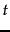, what would these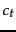numbers look like for the whole collection? odds-ratio-ct-contingency gives a contingency table of counts of documents in the collection, where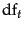is the number of documents that contain term: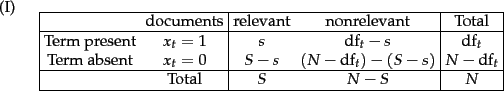Using this,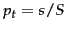and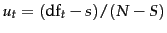and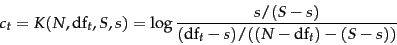(74)

To avoid the possibility of zeroes (such as if every or no relevant document has a particular term) it is fairly standard to add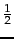to each of the quantities in the center 4 terms of odds-ratio-ct-contingency, and then to adjust the marginal counts (the totals) accordingly (so, the bottom right cell totals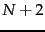). Then we have: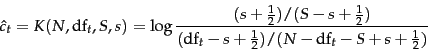(75)

Addingin this way is a simple form of smoothing. For trials with categorical outcomes (such as noting the presence or absence of a term), one way to estimate the probability of an event from data is simply to count the number of times an event occurred divided by the total number of trials. This is referred to as the relative frequency of the event. Estimating the probability as the relative frequency is the maximum likelihood estimate (or MLE ), because this value makes the observed data maximally likely. However, if we simply use the MLE, then the probability given to events we happened to see is usually too high, whereas other events may be completely unseen and giving them as a probability estimate their relative frequency of 0 is both an underestimate, and normally breaks our models, since anything multiplied by 0 is 0. Simultaneously decreasing the estimated probability of seen events and increasing the probability of unseen events is referred to as smoothing . One simple way of smoothing is to add a number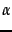to each of the observed counts. These pseudocounts correspond to the use of a uniform distribution over the vocabulary as a Bayesian prior , following Equation 59. We initially assume a uniform distribution over events, where the size ofdenotes the strength of our belief in uniformity, and we then update the probability based on observed events. Since our belief in uniformity is weak, we use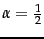. This is a form of maximum a posteriori ( MAP ) estimation, where we choose the most likely point value for probabilities based on the prior and the observed evidence, following Equation 59. We will further discuss methods of smoothing estimated counts to give probability models in Section 12.2.2 (page); the simple method of addingto each observed count will do for now.Next: Probability estimates in practice Up: The Binary Independence Model Previous: Deriving a ranking function   Contents   Index